## Introduction

Quantum mechanics is one in a large class of theories which are consistent with relativity in the sense that they do not allow signals to be sent faster than the speed of light. Many of these theories exhibit strong non-local correlations between distant particles that cannot be explained by the properties of the individual particles alone. Surprisingly, quantum mechanics is not the most non-local among them, which raises the question about the physical principle that singles out quantum mechanics and sets the limit on the possible strength of correlations in nature.

Here we experimentally address this fundamental question by testing the principle of information causality in the classical, quantum and post-quantum regime. While the no-signaling principle limits the speed with which distant parties can communicate, information causality states that the accessible information cannot be more than the information content of a communicated message, no matter what other shared resources are used. Both classical and quantum mechanics satisfy this principle, while it is violated by most post-quantum theories1.

We experimentally emulate correlations of various strengths from classical to almost maximally non-local and demonstrate a violation of the principle of information causality in the case where the simulated correlations are beyond the quantum regime. Apparent super-quantum correlations are, in our approach, a consequence of the non-unitary evolution of quantum states when subjected to polarization-dependent loss with post-selection2. For moderate loss, we find that initially entangled states can result in super-quantum correlations, while unentangled states still appear classical. For higher loss on the other hand we observe super-quantum correlations even for classical input states.

No-signaling resources can formally be treated as pairs of black boxes shared between arbitrarily separated Alice and Bob3, see Fig. 1a). Each box has a single input and output and the correlation between them is only restricted by the no-signaling principle. This means that the local outcome only depends on the local input, such that Alice cannot learn anything about Bob's input from only her output.

A typical quantum example of such a resource is a pair of entangled particles, shared between Alice and Bob, where inputs correspond to measurement settings and outputs to measurement outcomes. Since the work of John Bell—and numerous subsequent confirming experiments—it is now widely accepted that these particles exhibit non-local correlations, which have no classical explanation. Under the no-signaling constraint alone, however, there are even stronger non-local correlations than quantum entanglement4. The maximum that is compatible with relativity is achieved by the so-called Popescu-Rohrlich (PR)-box4, characterized by perfect correlations of the form AB = ab, between Alice's and Bob's inputs a and b and outputs A and B, respectively. Here denotes addition modulo 2, equivalent to the logical XOR, where AB = 0 when A = B and 1 otherwise.

A convenient operational way of quantifying non-locality is the Clauser-Horne-Shimony-Holt (CHSH) inequality5. This experimentally testable reformulation of Bell's inequality is satisfied by any correlation that can be described by a local hidden variable model. Such models are a description of correlations that can arise in classical systems, but cannot describe non-local correlations obtained from e.g. entangled quantum states. Written in terms of correlations of the form A B = ab the inequality takes the form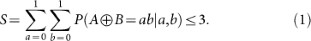Here P(A B = ab | a, b) denotes the probability for obtaining outputs A, B, which satisfy A B = ab given the inputs a for Alice and b for Bob. While this inequality is satisfied by any classical correlations, it can be violated in the quantum case. This violation, however, is bounded to a value of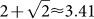, known as Tsirelson's bound6. Note, that inequality (1) is presented here in a slightly different form than conventionally5, where the classical bound is 2 and Tsirelson's bound is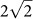. They are, however, linearly related and the difference is a simple rescaling of S.

Despite being a simple consequence of the mathematical formalism of quantum mechanics, it is unclear what the physical motivation is for this seemingly sub-optimal limit on the strength of quantum correlations. In fact even the algebraic maximum S = 4 can be achieved (by the PR-box) without violating the no-signaling principle.

This principle is physically motivated by the fact that, according to special relativity, faster-than-light information transfer would allow information to be sent backwards in time and thus violate causality. Nevertheless, it does not explain why super-quantum correlations such as the PR-box are incompatible with quantum mechanics and seem not to exist in nature. A possible explanation is offered by the principle of information causality—a generalization of no-signaling—which states that there cannot be more information available than was transmitted7.

This can be understood on the basis of the following elementary information-theoretic protocol: Bob tries to gain information from a set of data that is only known to Alice. The parties are allowed to use an arbitrary amount of shared no-signaling resources, but may not communicate more than m classical bits. In this case, the information causality principle states that the amount of information accessible to Bob should be limited to m classical bits7.

In the simplest instance, Alice has a set of two bits {a0, a1} and Bob wants to guess one of them, which we denote ab8, see Fig. 1a). Alice and Bob then input a0 a1 and b into their respective black box and obtain outputs A and B. From this output Alice computes an m = 1-bit message M = Aa0 and sends it to Bob, who calculates his guess for Alice's bit as G = MB = a0 A B. In the case of a shared PR-box, Bob can guess either one of Alice's bits perfectly, since in that case A B = ab and thus G = a0 b(a0 a1).

In the more general case considered here, Alice has a dataset {a0, … aN−1} of N = 2n bits and Bob wants to guess the bit with index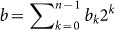. As discussed in Ref. 7, Alice and Bob can achieve this task by using a nested version of the protocol outlined above, with N − 1 black boxes on n levels and 1 bit of classical communication.

The protocol is illustrated in Fig. 2b) for the case n = 2. From every output Alice computes a temporary message Mk,i, where k denotes the level and i the number of the box on that level. Since she is only allowed 1 bit of communication, she uses these temporary messages as the inputs for the boxes on the next-lower level and only sends the final message to Bob. Depending on bn Bob then decodes either Mn−1,1 or Mn−1,2 and then moves on to the next-higher level until he reaches the bit of interest.

Bob's success can then be quantified by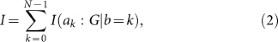where I(ak : G | b = k) is the Shannon mutual information between the k'th bit of Alice's list and Bob's guess for it7. This quantity can further be bounded as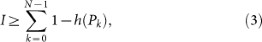where h(Pk) is the binary entropy of the success probability Pk for guessing the k'th bit.

## Results

Experimentally, we generate apparent super-quantum correlations based on the effect of polarization-dependent loss in a post-selected Bell-test experiment2, see Fig. 2a). We use photon pairs created from a continuous-wave pumped spontaneous parametric down-conversion source in a polarization Sagnac design9,10, as illustrated in Fig. 2b). Using this approach we obtain photon pairs with very high efficiency and in a continuously tunable fashion that enables us to produce any bipartite quantum state11.

In particular, we used the maximally entangled state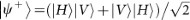as the initial state, where |H/V〉 represent horizontal and vertical polarization, respectively. For comparison, we also considered the corresponding fully decohered and thus separable state ρsep = (|HV〉〈HV| + |V H〉〈V H|)/2. This state was produced as a mixture of the two pure state components |HV〉 and |V H〉 by probabilistically mixing the respective coincidence counts.

The initial state is then subjected to polarization-dependent loss, introduced to the system by means of a Jamin-Lebedev polarization-interferometer, which allows individual control of the degree of loss for each polarization mode for both Alice and Bob, see Fig. 2c). In the symmetric case considered here the loss was parametrized by a single parameter κ, where κ = 0 corresponds to the loss-free scenario and κ = 1 means complete loss of one polarization. With this setup we simulated correlations of increasing strength, ranging from classical to quantum and close to maximal non-signaling as discussed in detail in the methods section.

Using these correlations we investigated the information causality protocol on up to four levels (corresponding to a 16-bit data-set for Alice) with 1-bit of communication. Crucially, we implemented the protocol in Fig. 1b) on a shot-by-shot basis, rather than estimating the performance from coincidence probabilities. For this we used an AIT-TTM8000 time-tagging module with a temporal resolution of 82 ps to register the single photon counts for all possible outcomes. From this data, using passive feed-forward, i.e. at the processing stage, we were able to reconstruct over 105 individual trials of the protocol for each of the 21 settings of uniformly increasing κ.

At a correlation strength of S = 3.874(5), the information available to Bob is at least I ≥ 1.86(2) bits, despite only receiving 1 bit from Alice. For four nesting levels of the protocol we establish lower bounds as high as I ≥ 7.47(11) bit, which violates the information causality inequality I ≤ 1 by almost 60 standard deviations. Similarly for weaker correlations, Bob has more information available than contained in Alice's message for all nesting levels as soon as the correlation strength surpasses S ≈ 3.5. The fact that this value is significantly higher than Tsirelson's bound of SQ ≈ 3.41 emphasizes that the quantity I only recovers this bound in the asymptotic limit n → ∞.

In the following we therefore consider an alternative figure of merit, motivated by identifying the protocol in Fig. 1b) as a special case of a so-called random access code12. Using similar ideas as in Ref. 7, the efficiency of this task can be bounded by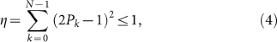which thus also encompasses the principle of information causality12. This bound, however, can indeed be saturated by quantum states for any size of Alice's dataset, as illustrated in Fig. 3. Note that our data violates the bound before the correlations surpass Tsirelson's bound. This is a result of a slight anisotropy in the simulated correlations due to experimental imperfections and a resulting bias for certain data-sets. It is not present when considering isotropic correlations, see Fig. 3b). Crucially, this highlights the dependence of both figures of merit (3) and (4) to the specific random choice of Alice's data-set.

In particular, the separable state used in the simulation produces entanglement-like correlations for one measurement choice of Alice and uncorrelated outputs for the other, see Supplementary Figure S1. Hence, depending on the choice of data-set the figures of merit η and I might resemble the behavior expected for an entangled state, for a completely mixed state or, for higher nesting level, anything in-between. Only when averaging over all possible datasets, {ai}, for a given level or employing the “depolarization” protocol introduced in Ref. 13 to make the correlations isotropic without changing the CHSH value, can the quantities (3) and (4) be used as reliable figures of merit, see Supplementary Fig. S1 and S2. Note, however, that anisotropic super-quantum correlations (averaged over all datasets) do not necessary violate Tsirelson's bound. In this case the principle of information causality cannot be probed using the depolarization approach, since it would result in isotropic correlations and information causality would not be violated.

## Discussion

In contrast to the full set of no-signaling correlations and the set of classical correlations, which both have the form of a well-characterized polytope, much less is known about the quantum set3,14. Understanding the set of quantum correlations theoretically and characterizing it experimentally should thus be a primary aim from a practical as well as a fundamental perspective. Information causality, which has been proposed as a physical principle to reconstruct the set of quantum correlations, has already proven successful in recovering the famous Tsirelson bound. This limit of quantum correlations, however, is only one extremal point on the continuous boundary and there exist correlations below it, which nevertheless do not admit a quantum description1. Information causality also rules out such correlations for some 2-dimensional slices of the full (8-dimensional) no-signaling polytope, while it does not for other slices1. This shortcoming, nevertheless is not definite and might just be a result of a suboptimal protocol in Fig. 1b).

A violation of information causality would in particular imply that the tested theory does not admit a suitable measure of one of the most elementary information theoretic quantities: entropy12,15. Such a measure is assumed to be consistent with the classical limit and such that the entropy change ΔH of a composite system XY satisfies ΔH(XY) ≥ ΔH(X) + ΔH(Y) under local evolution of the subsystems X and Y. Hence, a failure of these requirements could be interpreted as allowing for the generation of non-local correlations via local transformations. Similar consequences might also arise from the violation of alternatives to information causality, which are more or less successful in recovering part of the quantum boundary. Examples include the principles of local orthogonality16, the requirement that the theory has a suitable classical limit17 or that certain communication18,19,20 or computational tasks21 are non-trivial.

Our method of simulating super-quantum correlations could be adapted to explore some of these alternative principles as well. Of particular interest, however, would be a test of information causality in the multipartite case, since most of the above principles are formulated in the bipartite setting, which is bound to fail in recovering the full quantum boundary due to the existence of multipartite super-quantum correlations, which obey every bipartite principle22,23. While there are studies of information causality for higher-dimensional systems, which strengthen its position as a physical principle that determines quantum correlations24, a suitable generalization to the multipartite case is still an open problem.

As highlighted by our experiment, special focus has to be put on anisotropic regions of the no-signaling polytope. Specifically we find that the introduced figures of merit are not valid in a single instance of the protocol and have to be averaged over all possible datasets or estimated from the depolarized, isotropic data. This subtle, but very important detail is clearly highlighted by our experimental results, where we show how even a small amount of imbalance can result in a violation of the principle by quantum states for a specific choice of parameters, while obeying the principle on average.

## Methods

Examining the results of a CHSH-inequality test make it clear where our data crosses the boundary of the quantum set. In our investigation we focused on the scenario of a fixed maximally entangled state |ψ+〉 in situations with different amounts of loss, as shown in Fig. 4. We further considered the state ρsep, which resembles the state |ψ+〉 after full decoherence as might happen during propagation between Alice and Bob. This allows for an intuitive comparison between the entangled and unentangled case.

The tested inequality has the form of a CHSH-inequality with measurements in the yz-plane of the Bloch sphere. For the lossless case κ = 0, Alice's and Bob's measurements can be viewed as the application of appropriate basis-rotations (around the X-axis) followed by projective measurements in the |H/V〉-basis. These rotations can also be seen as phase-gates in the diagonal polarization basis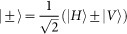. In the case where polarization dependent loss is present, these phase-gates become non-unitary. They act as the identity on the state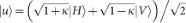and impose a phase on the non-orthogonal state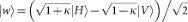, where κ = 〈u|w〉. The precise relation between κ and the degree of loss is discussed in Ref. 2. As non-unitary operations can only be performed non-deterministically, postselection on success is required, which results in the observation of apparent super-quantum correlations. Finally, we use the first step of the depolarization procedure in Ref. 13 to symmetrize the simulated correlations, while preserving their possible anisotropy.

Curiously, we note that moderate polarization-dependent loss can lead to super-quantum correlations for entangled states without invalidating the CHSH inequality for separable states, as suggested in Ref. 2. This observation even holds when optimizing the separable state for maximal CHSH-value, for each degree of loss2. Note, however, that these results were obtained using the same measurements for both separable and entangled states, whereas arbitrary hidden variable theories would allow arbitrary measurements.

Figure 2 illustrates the obtained values of the CHSH parameter S and compares them to the ideal case, which, for the initially entangled state, is described by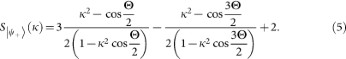Here Θ is a function of κ, which can be analytically approximated by Θ = π(17+ cos(πκ))/12, as discussed in Ref. 2.

We experimentally violate Tsirelson's bound by more than 7 standard deviations, S = 3.423(1) at a loss parameter of κ = 0.3. At this point, the achieved value for the unentangled state, S = 2.821(2), is indeed well below the classical bound of 3 and even the optimal unentangled state does not violate the inequality until κ ≈ 0.37. In the region 0.3 ≤ κ ≤ 0.37 it is therefore possible to exploit super-quantum correlations from entangled states while unentangled states still appear classical. With increasing loss, both states eventually violate Tsirelson's bound and approach the numerical maximum of S = 4, with experimental values of S = 3.9341(6) and S = 3.929(2) for the entangled and separable state, respectively. The increasing deviation from the theoretical predictions in Fig. 4 is a result of the decreasing signal-to-noise ratio in the single-photon detectors for high-loss settings.

Related experiments have observed apparent violations of Tsirelson's bound as a consequence of explicit violations of the detection loophole25 or the fair-sampling assumption26. The latter is in fact typically violated if the quantum system of interest has more (possibly) correlated degrees of freedom than those tested in the Bell-inequality27. Violation of Tsirelson's bound has also been considered as an intermediate step in deriving three-qubit inequalities28.